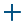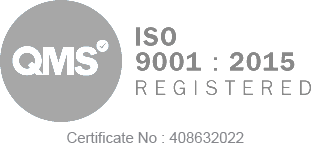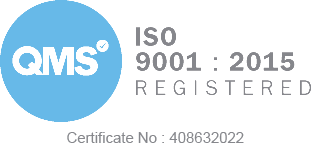# Mathematics and Statistics for IT

Computer Science and IT

(
IT402
)
R/617/6693
Level 4
£290
£1490
BLOCKCHAIN-BASED
R/617/6693
Level 4
£290
£1490
###### Verification
BLOCKCHAIN-BASED
Accelerate your career with London Institute of Business & Technology's flexible executive pathway programmes today!
##### Total Fee £1600

£2360 (£1600 during the tuition waiver period)

Please see the Tuition Fee section to see if you are eligible for a scholarship.
###### Course Overview

This course aims to provide an opportunity to learn mathematics and statistics and equip learners with the mathematical skills to analyse and solve problems that will enable them to work within the field of IT. The course covers number systems, logic, relations, functions, quadratic equations, quadratic functions, simultaneous equations, polynomial equations, exponential functions, logarithmic functions, coordinate geometry and matrices.

###### Course ContentIntroduction to the module

Statistics

• Introduction to Statistics
• Importance of Statistics.
• Scope of Statistics
• Career Opportunities
• Introduction to Population and Sample
• Collection of data.
• Primary and Secondary Sources

Frequency Distribution

• Introduction
• Univariate Frequency Distribution.
• Bivariate Frequency Distribution
• Graphs of Frequency Distributions
• Frequency Distributions and their Comparison

Probability

• Introduction to Probability
• Definition
• Formula
• Types of Probability
• Events and Set theory
• Probability Theory
• Application of Probability

Conditional Probability

• Definition
• Conditional Probability of Independent Events
• Conditional probability of Mutually Exclusive Events
• Properties of Conditional Probability
• Example
• Independent events
• Bayes’ theorem

Permission and Combinations

• Introduction
• Permutations
• Types of Permutation
• Combinations
• Relation between Permutation and Combination
• Difference between permutation and combination

The Binomial, Poisson and Normal distribution

• Binomial Distribution
• Poisson Distribution
• Normal Distribution
• Hypothesis Testing

Measures of Dispersion and Skewness

• Measures of Dispersion
• Methods of measuring dispersion
• Measures of Skewness
• Coefficient of variation

Correlation and Regression

• Introduction
• Karl Pearson’s coefficients of correlation
• Regression Analysis
• Properties of regression coefficients
• Curvilinear Regression
###### Learning Outcome & Assessment Criteria1.  Understand the mathematics underpinning information technology

1. Explain the nature of the roots of quadratic equations, the rules of exponents and logarithms and a function
2. Explain the relationship between a domain, range and function
3. Rewrite an exponential equation in logarithmic form and a logarithmic equation in exponential form
4. Compute maximum and minimum values of quadratic functions, composite functions, inverse functions, the area of a polygon, the equation of a straight line, locus, measures of central tendency and measures of dispersion and probability
5. Analyse the impact of quadratic inequalities, polynomial equations, exponential equations, logarithmic equations and simultaneous equations on hardware design

2.  Understand the statistics underpinning information technology

1. Calculate summary measures correctly
2. Define and interpret probability models
3. Evaluate methods of estimation and hypothesis testing
4. Analyse the concepts of statistical methodologies
###### Assessment• The assessment strategy for this course is designed to test all the learning outcomes.
• Learners must demonstrate successful achievement of all learning outcomes to pass the course.
###### Assessment Marking CriteriaScholarships

Citizens of the following countries are eligible for a 50% scholarship upon writing a personal statement. All scholarships are to be approved by the Academic Board. The list of countries are: Sri Lanka, Indonesia, Philippines, Bhutan, Morocco, Vietnam, Papua New Guinea, Laos, Cambodia, India, Nigeria, Ghana, Bangladesh, Laos, Myanmar, Pakistan, Nepal and South Africa. Please talk to your student counselor and ask for the Coupon Code to get the 50% Tuition Waiver.

• The assessment criteria for a pass will be presented with the summative exercise.
• Further information and guidance on the assessment will be made available through the tutor.
###### Unit Pass RequirementsTo pass the unit a 40% overall grade must be achieved.###### What is included in the cost of my programme?
• All course material, including online modules and written assignments
• Unlimited personal tutor support with online sessions
• Dedicated student support
• Assignment marking and feedback
###### Am I eligible for this programme?

You are eligible if you meet our stipulated entry requirements.

### Easy Monthly Payments

£155
/monthEasy 12 instalmentsComplete in 12 monthsUnlimited tutor supportRequires submitting 1 assignment per month

MOST POPULAR

### Easy Monthly Payments

£290
/monthEasy 6 instalmentsComplete in 6 monthsPriority supportUnlimited tutor supportRequires submitting 2 assignments per month

### FLEXI MODE

£1,600
1-timeNo monthly paymentsPriority supportUnlimited tutor supportDedicated counselor